Statics

Introduction

Statics is all about objects that are stationary. We're concerned with making sure objects stay in equilibrium by resolving forces.

Weight and gravity

The earth's gravitational field exerts a force on you at all times, causing objects to fall towards the earth if dropped from some height.

In accordance with N2L this force causes an acceleration due to gravity, denoted $g$. In M1 $g$ is taken to be constant at $9.8\textrm{ms}^{-2}$.

A consequence of this is that you have a weight, the force required to accelerate you towards the earth at $g$.

$$\textrm{weight} \left(\textrm{N}\right) = \textrm{mass} \left(\textrm{kg}\right) \times \textrm{acceleration due to gravity} \left(\textrm{ms}^{-2}\right)$$

In our notation

$$W = mg$$

Free body diagrams

A free body diagram (FBD) allows you to analyse the magnitudes and relative directions of all the forces acting on an object.

The diagram consists of the object and all the forces it is being subjected to. We mark down the directions of these forces and (if we know them) their magnitudes.

Here's an example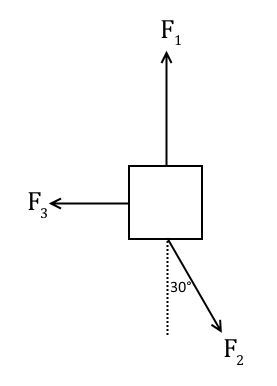From there you can resolve all the different forces in each direction to check it will stay in equilbrium.

Resolving forces

You need to resolve forces in a certain direction to ensure that there is equilibrium in that direction, or to calculate if there is a resultant force.

Note that forces that are orthogonal (90° to each other) cannot be compared. When resolving in one direction, we only take into account the forces and force components that act in that direction.

Forces are not always exerted in line with each other, but they have components in certain directions that can be compared with other forces. Take the force $F$ at an angle $\theta$ to some line. It can be resolved into components parallel to the line and orthogonal to the line.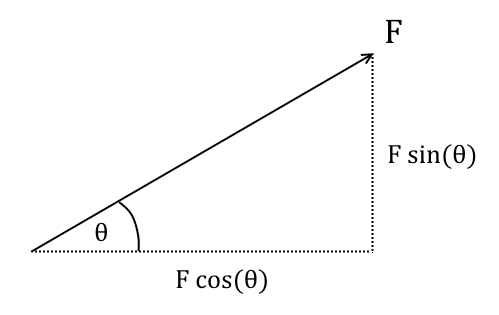In M1 you will deal with a lot of right angle triangles. To make it easier on yourself, remember the following rules for $\sin\theta$ and $\cos\theta$ with $\theta$ in degrees

$$\sin(90^{\circ}-\theta) = \cos\theta$$

$$\cos(90^{\circ}-\theta) = \sin\theta$$

Example

Q) A 4kg ball is placed on a smooth slope inclined at 30° to the horizontal. A force $P$ is applied to the ball parallel to the line of the slope. Find the required magnitude of $P$ so that the ball does not roll down the slope.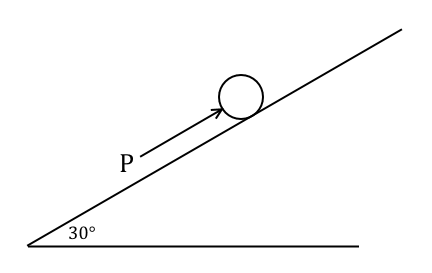A) For the ball to be in equilbrium we need the resultant of the forces acting parallel to the slope to be zero. Note that the ball's weight acts vertically downwards and has magnitude $mg = 4g\textrm{N}$. It is 90°-30° = 60° from the line of the slope.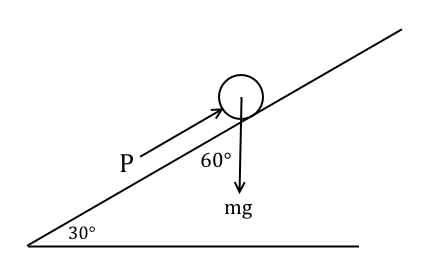Therefore for equilbrium up the slope we need

$$P - 4g \cos(60^{\circ}) = 0$$ $$\therefore P = 4g\cos(60^{\circ}) = 19.6\textrm{N}$$

Example

Q) A 4kg spherical light is held in place by a 2.8m long wire attached to the side of a building, so that the light is 2m horizontally from the building. Find the tension in the wire.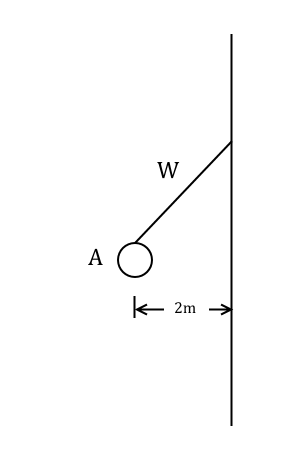A) We need to resolve forces acting on the light, remembering that the light is held in equilibrium. The light's weight is $4g = 39.2 \textrm{N}$ and we'll label the tension in $W$ as $T$. The wire is also diagonal relative to the weight of the light, so we'll need to take that into account when resolving forces.We need to find $\theta$ first by using trigonometry. We're told that the length adjacent to $\theta$ is 2m, and the hypotenuse (the length of the wire) is 2.8m. Therefore $\cos\theta = \frac{2}{2.8} \Rightarrow \theta = \cos^{-1}\left(\frac{2}{2.8}\right) = 44.4^{\circ}$ (3.s.f.).

So the free body diagram for the light looks like this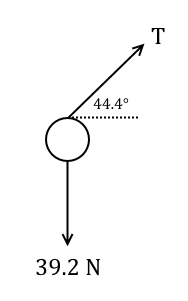To find the tension in the wire $T$ we need to resolve vertically. For there to be equilibrium there must be no resultant force.

$$T \sin(44.4^{\circ}) - 39.2 = 0$$

$$\therefore T = \frac{39.2}{\sin(44.4^{\circ})} = 56.0 \textrm{N} \left(\textrm{3.s.f.}\right)$$

Example

Q) A 3.5kg sphere is held in place by two wires attached to a ceiling. Given that the tension in the right hand wire is 23N, find the tension in the left hand wire.A) The sphere's weight is $3.5g = 34.3\textrm{N}$. Labelling the left hand wire's tension as $T_1$ and the right hand wire's tension as $T_2 = 23 \textrm{N}$, we amend the diagram with these forces.We can resolve forces on the sphere vertically, remembering that the system must be in equilbrium.

$$T_1\sin(40^{\circ}) + 23\sin(68^{\circ}) - 34.3 = 0$$

$$\therefore T_1 = \frac{34.3-23\sin(68^{\circ})}{\sin(40^{\circ})} = 20.2 \textrm{N} \left(\textrm{3.s.f.}\right)$$

Friction

In real life surfaces aren't always perfectly smooth. Friction is the resistive force that acts against objects sliding across surfaces. It is a consequence of Newton's third law of motion. In M1 surfaces have a coefficient of friction, a number between 0 and 1, which determines how 'rough' the surface is, i.e. how much it resists motion. It is denoted $\mu$. A surface with $\mu = 0$ is perfectly smooth, and a surface with $\mu = 1$ is perfectly rough.

The coefficient of friction of a surface relates the friction force $F_f$ experienced by an object sliding across it, and the normal reaction force $R$ perpendicular to the surface due to the object's weight and other external forces.

$$F_f = \mu R$$

The friction force $F_f$ and reaction force $R$ are always orthogonal. If you think about it this is obvious.

Friction force always acts in the direction opposite to that of motion. But since we are talking about static objects in equilbrium, we can find the direction of the friction force by saying that an object is 'on the point of moving' upwards or downwards.

That means that the object is on the verge of moving in the given direction, and when you resolve forces you know to draw friction acting in the opposite direction.

When drawing force diagrams I sometimes draw friction closer to the ground, but that's up to you.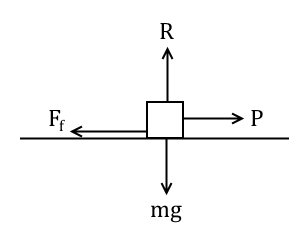Example

Q) A 1kg particle is held in equilbrium on a rough slope by a force $P$ of magnitude 10N applied horizontally. If the particle is on the point of moving up the plane, and $\sin\theta = \frac{3}{5}$, find the coefficient of friction and the magnitude of the friction on the particle.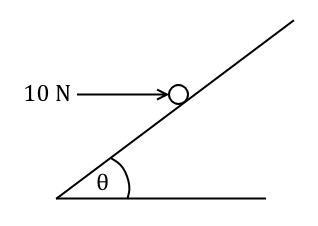A) The weight of the particle is $g = 9.8 \textrm{N}$ and the horizontal force $P = 10\textrm{N}$. We're also given that $\sin\theta = \frac{3}{5}$. Therefore from the simple 3-4-5 triangle we know that $\cos\theta = \frac{4}{5}$.

We're also told that the particle is on the point of moving up the slope, so take friction as pointing down the slope. We can now amend the diagram with the forces that we know of.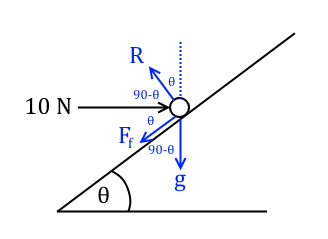Taking horizontal equilibrium we get

$$F_f \cos\theta + R \sin\theta - 10 = 0$$

$$\Rightarrow \frac{4}{5}F_f + \frac{3}{5}R = 10$$

And taking vertical equilibrium we get

$$R \cos\theta - F_f \sin\theta -g = 0$$

$$\Rightarrow \frac{3}{5}F_f - \frac{4}{5}R = -g$$

We now have the simultaneous equations

\begin{align} \frac{4}{5}F_f + \frac{3}{5}R &= 10 \\ \frac{3}{5}F_f - \frac{4}{5}R &= -g \end{align}

Solving them yields $F_f = 2.12\textrm{N}$ and $R=13.84\textrm{N}$, therefore $\mu = \frac{2.12}{13.84} = 0.153$ (3.s.f.).